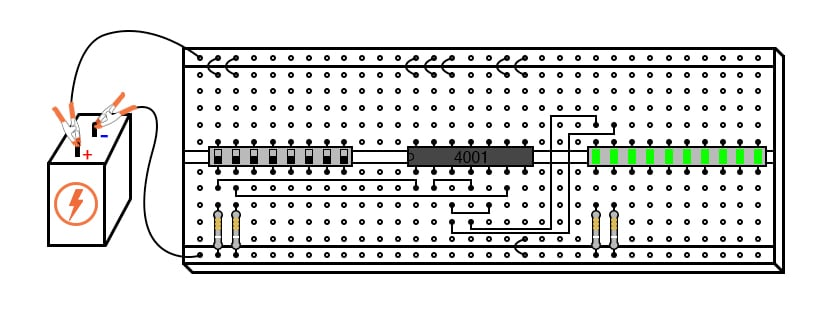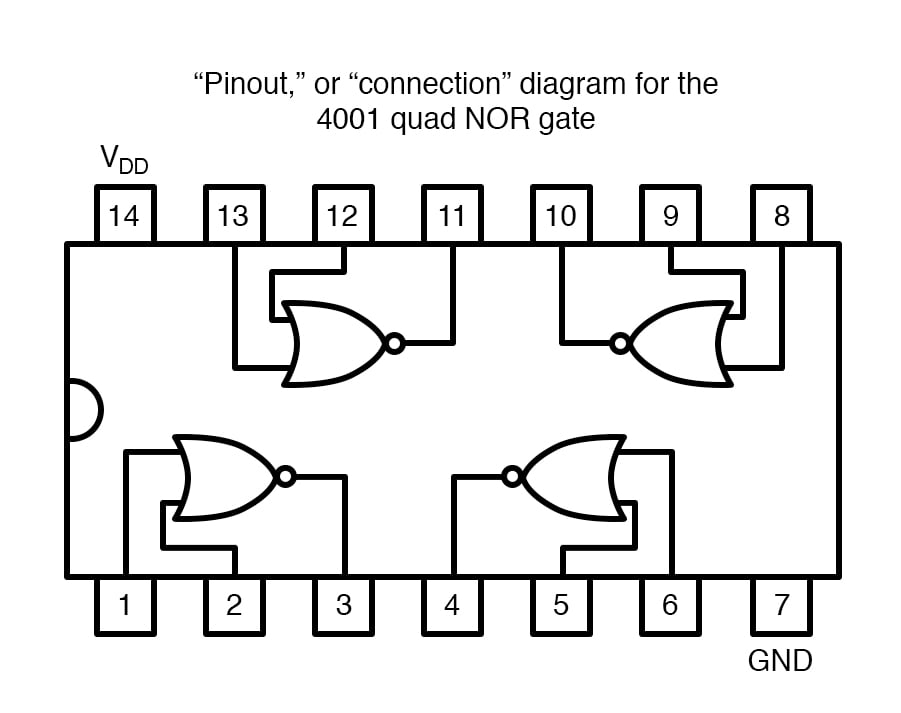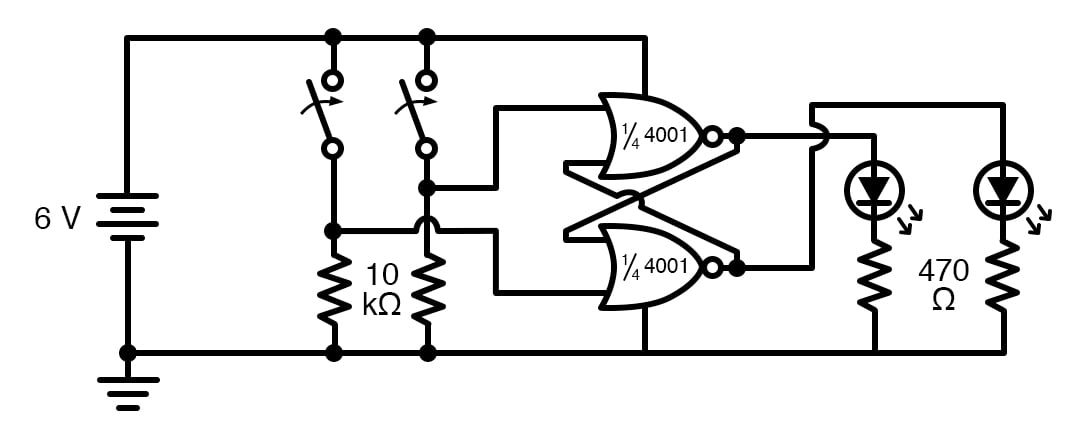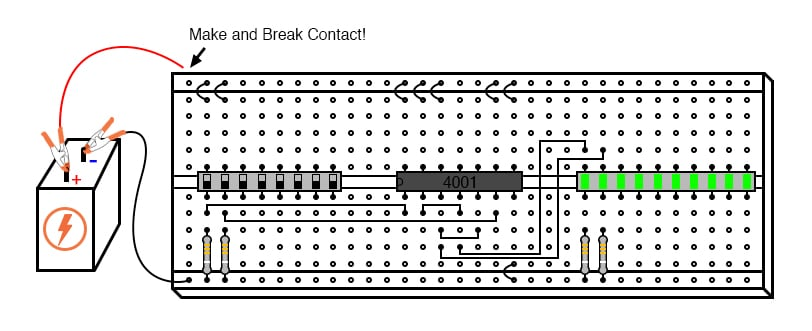Vol. DIY Electronics Projects
Chapter 7 Digital IC Projects

# Digital Lab - S-R Latch Using NOR Gates

## In this hands-on electronics experiment, build an S-R latch using two NOR gates and learn how this type of digital memory operates, including invalid states, race conditions, and the effects of output loading.

### Project Overview

S-R latches are a fundamental digital logic circuit that performs a memory function that allows it to store a logical 0 or 1. In this project, you will build and test the S-R latch circuit with switch inputs and light-emitting diode (LED) outputs shown in Figure 1.##### Figure 1. S-R latch with switch inputs and LED outputs.

In the first portion of the project, you will examine the standard operation of the S-R latch. Then, you will use this simple circuit to learn about some unique aspects of digital circuits, including invalid logic states, race conditions, and output loading.

### Parts and Materials

• Eight-position dual inline package (DIP) switch
• Ten-segment bar graph LED
• One 6 V battery
• Two 10 kΩ resistors
• Two 470 Ω resistors
• Two 100 Ω resistors

Caution! The 4001 IC is a complementary metal-oxide semiconductor (CMOS) and, therefore, sensitive to static electricity!

### Learning Objectives

• To learn the effects of positive feedback in a digital circuit
• What is meant by the invalid state of a latch circuit
• What a race condition is in a digital circuit
• To know the importance of valid high CMOS signal voltage levels

### Instructions

Step 1: Review the pinout diagram of the 4001 CMOS quad NOR gate integrated circuit, illustrated in Figure 2.##### Figure 2. Pinout package diagram for the 4001 quad NOR gate

It is identical in input, output, and power supply pin assignments to the 4011 quad NAND gate.

#### Build the S-R Latch with LED Output Indicators

Step 2: Cross-connect two NOR gates, as shown in the schematic diagram of Figure 3, on a breadboard as illustrated in Figure 4.##### Figure 3. Schematic diagram of an S-R latch built using NOR gates##### Figure 4. Breadboard implementation of an S-R latch built using NOR gates

This cross-connection creates positive feedback from output to input. That is, the output signal tends to maintain the gate in its last output state. Just as in op-amp circuits, positive feedback creates a hysteresis. This tendency for the circuit to remain in its last output state gives it a sort of memory. In fact, there are solid-state computer memory technologies based on circuitry like this!

Step 3: Connect two of the switches to the remaining NOR gate inputs, and add a 10 kΩ pulldown resistor to each switch (Figures 3 and 4).

Step 4: Connect the outputs of the two NOR gates to LEDs and add 470 Ω resistors in series with the LEDs to limit the currents. If we designate the left switch as the SET input and the right switch as the RESET input, the left LED will be the Q output and the right LED the $$\overline{Q}$$ output. $$\overline{Q}$$ is the logical inverse of Q and is referred to as "Q-not."

$$\overline{Q} = \neg Q = ~!Q$$

#### Test the S-R Latch Operation

Step 5: Now, switch the SET input high (switch on for logic 1) and the RESET input low (switch off for logic 0). Q will go high (1) and turn on its LED, while $$\overline{Q}$$ will go low (0) to turn off its LED. This is known as the set state of the S-R latch.

Step 6: Making the RESET input high (1) and the SET input low (0) reverses the latch circuit’s output state: Q low (0) and $$\overline{Q}$$ high (1). This is known as the reset state of the circuit.

Step 7: Place both inputs into the low (0) state. The circuit’s Q and $$\overline{Q}$$ outputs will remain in their last states, remembering their prior settings. This is known as the latched state of the circuit.

Step 8: Since the outputs have been designated Q and $$\overline{Q}$$, it is implied that their states will always be complementary (opposite). Thus, if something were to happen that forced both outputs to the same state, we would be inclined to call that mode of the circuit invalid. This is exactly what will happen if we make both SET and RESET inputs high: both Q and $$\overline{Q}$$ outputs will be forced to the same low (0) logic state.

Give it a try. This is known as the invalid or illegal state of the circuit, not because something has gone wrong but because the outputs have failed to meet the expectations established by their labels.

Step 9: Since the latched state is a hysteretic condition whereby the last output states are remembered, one might wonder what will happen if the circuit powers up this way with no previous state to hold. To experiment, place both switches in their off positions, making both SET and RESET inputs low, then disconnect one of the battery wires from the breadboard, as shown in Figure 5.#### Examining Race Conditions in the S-R Latch Circuit

Step 10: Quickly make and break contact between that battery wire and its proper connection point on the breadboard, noting the status of the two LEDs as the circuit is powered up repeatedly. When a latch circuit such as this is powered up into its latched state, the gates race against each other for control. Given the low inputs, both gates try to output high signals. If one of the gates reaches its high output state before the other, that high state will be fed back to the other gate’s input to force its output low, and the race is won by the faster gate.

Invariably, one gate wins the race due to internal variations between gates in the chip and/or external resistances and capacitances that act to delay one gate more than the other. What this usually means is that the circuit tends to power up in the same mode over and over again. However, if you are persistent in your powering/un-powering cycles, you should see at least a few times where the latch circuit powers up latched in the opposite state from normal.

Race conditions are generally undesirable in any system, leading to unpredictable operations. They can be particularly troublesome to locate, as this experiment shows, because of the unpredictability they create. Imagine a scenario, for instance, where one of the two NOR gates was exceptionally slow-acting due to a defect in the chip. This handicap would cause the other gate to win the power-up race every time. In other words, the circuit will be predictable on power-up with both inputs low. However, suppose that the unusual chip was to be replaced by one with more evenly matched gates or by a chip where the other NOR gate was consistently slower.

Normal circuit behavior is not supposed to change when a component is replaced, but if race conditions are present, a change of components may very well do just that. Due to the inherent race tendency of an S-R latch, one should not design a circuit with the expectation of a consistent power-up state but rather use external means to force the race so that the desired gate always wins.

Step 11: An interesting modification to try in this circuit is to replace one of the 470 Ω LED dropping resistors with a lower-value unit, such as 100 Ω. The obvious effect of this alteration will be increased LED brightness, as more current is allowed through. A not-so-obvious effect will also result, and it is this effect that holds great learning value. So, try replacing one of the 470 Ω resistors with a 100 Ω resistor, and operate the input signal switches through all four possible setting combinations, noting the circuit's behavior.

You should note that the circuit refuses to latch in one of its states (either SET or RESET) but only in the other state when the input switches are both sets low (the latch mode). Why is this?

Step 12: Use a voltmeter to measure the output voltage of the gate whose output is high when both inputs are low. Note this voltage indication, then set the input switches so that the other state (either RESET or SET) is forced, and measure the output voltage of the other gate when its output is high. Next, note the difference between the two gate output voltage levels, one gate is loaded by an LED with a 470 Ω resistor, and the other is loaded by an LED with a 100 Ω resistor.

The one loaded down by the heavier load (100 Ω resistors) outputs a lower voltage. So much less voltage that it will not be interpreted by the other NOR gate’s input as a high signal at all as it is fed back! All logic gates have permissible high and low input signal voltage ranges, and if the voltage of a digital signal falls outside this permissible range, it might not be properly interpreted by the receiving gate.

In a latch circuit such as this, which depends on a solid high signal fed back from the output of one gate to the input of the other, a weak signal will not be able to maintain the positive feedback necessary to keep the circuit latched in one of its states. This is why I favor using a voltmeter as a logic probe for determining digital signal levels rather than an actual logic probe with high and low lights. A logic probe may not indicate the presence of a weak signal, whereas a voltmeter definitely will by means of its quantitative indication.

This type of problem, common in circuits where different families of integrated circuits are mixed (TTL and CMOS, for example), can only be found with test equipment providing quantitative signal level measurements.

### Related Content

Textbook:

Resistor Guide:

Worksheets:

#### Lessons in Electric Circuits

Published under the terms and conditions of the Design Science License